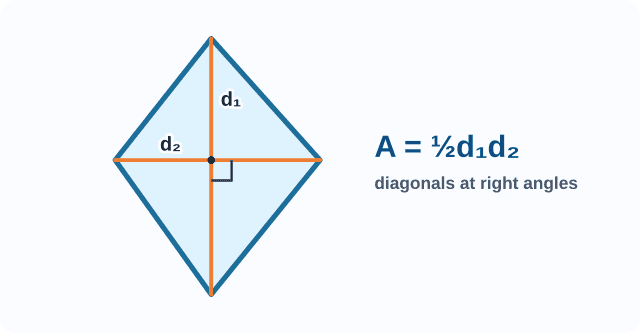index: click on a letter A B C D E F G H I J K L M N O P Q R S T U V W X Y Z A to Z index index: subject areas numbers & symbols sets, logic, proofs geometry algebra trigonometry advanced algebra & pre-calculus calculus advanced topics probability & statistics real world applications multimedia entrieswww.mathwords.com about mathwords website feedback

Area of a Kite

The area of a kite is half the product of the diagonals. Note: This formula works for the area of a rhombus as well, since a rhombus is a special kind of kite. Note that the diagonals of a kite are perpendicular.

 Kite d1 = long diagonal of kite d2 = short diagonal of kite Area = (½) d1d2